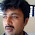## Friday, 24 May 2013

### CBSE Class 8 - Science - CH11 - Force and Pressure (MCQs)

Force and Pressure

(MCQs)

Q1: Opening a door is an example of ._______

(a) Contact force
(b) Non contact force
(c) Spring force
(d) Magnetic force

Q2: What is the cause of change in motion or change in the state of motion?

(a) Pressure
(b) Atmospheric Pressure
(c) Friction
(d) Force

Q3: A charged balloon attaches to a wall because of ______

(a) Muscular force
(b) Electrostatic force
(c) Magnetic force
(d) Gravitational force

Q4: The unit of force is _______

(a) Newton
(b) Dyne
(c) kg. weight
(d) All of these

Q5: What is force?

(a) Push
(b) Pull
(c) both a and b
(d) None of these

Q6: A body is said to be under balanced forces when the resultant force applied on that body is_____

(a) One
(b) Zero
(c) Infinite
(d) None of these

Q7: The ratio of force, acting perpendicular to the area, on which it acts is known as ________.

(a) Force
(b) Friction
(c) Presuure
(d) Density

Q8: The pressure of the water at the bottom of the pond is _______  at the surface of the pond.

(a) Lower than
(b) Higher than
(c) Same
(d) either lower or higher

Q9: As we go to the higher altitude the atmospheric pressure ________.

(a) Increases
(b) Decreases
(c) Remains same
(d) Cannot say

Q10: The force involved in falling an apple from a tree is:

(a) Magnetic force
(b) Contact force
(c) Electrostatic force
(d) Gravitational force

1: (a) Contact force
2: (d) Force
3: (b) Electrostatic force
4: (d) All of these
5: (c) both a and b
6: (b) Zero
7: (c) Presuure
8: (b) Higher than
9: (b) Decreases
10: (d) Gravitational force1.good questions

2.Pressure is not spelled 'pressure'

1.Then what it is spelled as ???
If it is not spelled 'pressure'

3.whats dyne?? i only know newton

1.unit for force haaa............

2.Unit of force is newton.{Q4}

3.it is unit of force
1 newton = 100000 dyne
dyne is a unit of force specified in the centimetre–gram–second system of units (CGS), a predecessor of the modern SI.

4.We can convert newton to kg etc.

4.5.Expecting more MCQs for this lesson

6.Very easy questions

7.i got full marks

8.i got full marks

9.SO EASY QUESTIONS

1.This comment has been removed by the author.

10.i also got full the questions are so easy

11.the 4 answer is wrong.. it is newton. checked in google. please refer another web page also.

1.In MKS systems and SI units, unit of force is Newton. In CGS system, it is dyne. Another unit used earlier to measure weights (a type of force) is kg-wt (1 kg-wt = 9.8N). Option 'd' is correct.

12.13.The SI unit of force is the newton,i checkout it on google and google is always right

14.15.Requesting 2 more mcq because have to do 12 in science notebook😂

16.Good questions but then it will be good if we get more tuff questions.

17.Nice yo🤘🏻🤘🏻